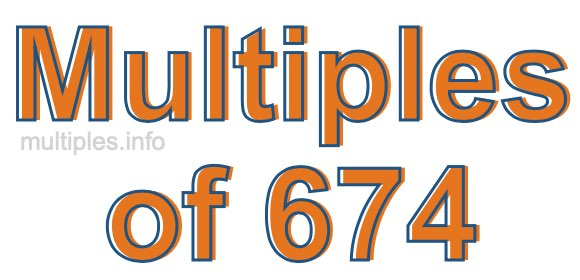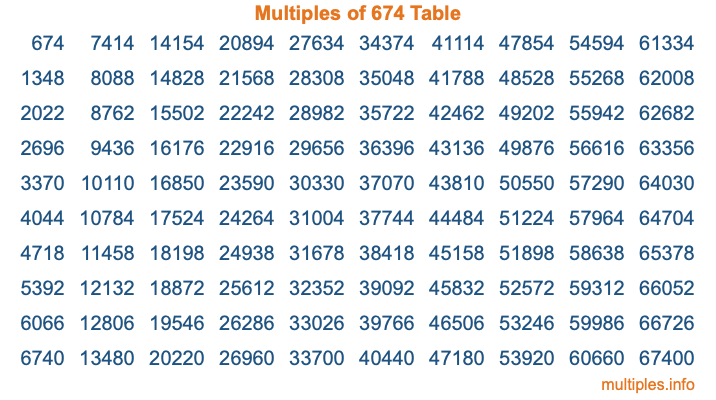Multiples of 674Welcome to the Multiples of 674 page. Here we will first teach you everything you will ever need to know about the multiples of 674, and then give you a study guide summary of everything we taught you to make sure you remember it all. Use this page to look up facts and learn information about the multiples of 674. This page will make you a multiples of six hundred seventy-four expert!

Definition of Multiples of 674
Multiples of 674 are all the numbers that when divided by 674 equal an integer. Each of the multiples of 674 are called a multiple. A multiple of 674 is created by multiplying 674 by an integer.

Therefore, to create a list of multiples of 674, you start with 1 multiplied by 674, then 2 multiplied by 674, then 3 multiplied by 674, and so on for as long as you want. Thus, the list of the first five multiples of 674 is 674, 1348, 2022, 2696, and 3370. To see a larger list of multiples of 674, see the printable image of Multiples of 674 further down on this page. We also have a category where you can choose any nth multiple of 674.

Multiples of 674 Checker
The Multiples of 674 Checker below checks to see if any number of your choice is a multiple of 674. In other words, it checks to see if there is any number (integer) that when multiplied by 674 will equal your number. To do that, we divide your number by 674. If the the quotient is an integer, then your number is a multiple of 674.

Is  a multiple of 674?

Least Common Multiple of 674 and ...
A Least Common Multiple (LCM) is the lowest multiple that two or more numbers have in common. This is also called the smallest common multiple or lowest common multiple and is useful to know when you are adding our subtracting fractions. Enter one or more numbers below (674 is already entered) to find the LCM.

Check out our LCM Calculator if you need more details about the Least Common Multiple or if you need the LCM for different numbers for adding and subtraction fractions.

nth Multiple of 674
As we stated above, 674 is the first multiple of 674, 1348 is the second multiple of 674, 2022 is the third multiple of 674, and so on. Enter a number below to find the nth multiple of 674.

th multiple of 674

Multiples of 674 vs Factors of 674
674 is a multiple of 674 and a factor of 674, but that is where the similarities end. All postive multiples of 674 are 674 or greater than 674. All positive factors of 674 are 674 or less than 674.

Below is the beginning list of multiples of 674 and the factors of 674 so you can compare:

Multiples of 674: 674, 1348, 2022, 2696, 3370, etc.

Factors of 674: 1, 2, 337, 674

As you can see, the multiples of 674 are all the numbers that you can divide by 674 to get a whole number. The factors of 674, on the other hand, are all the whole numbers that you can multiply by another whole number to get 674.

It's also interesting to note that if a number (x) is a factor of 674, then 674 will also be a multiple of that number (x).

Multiples of 674 vs Divisors of 674
The divisors of 674 are all the integers that 674 can be divided by evenly. Below is a list of the divisors of 674.

Divisors of 674: 1, 2, 337, 674

The interesting thing to note here is that if you take any multiple of 674 and divide it by a divisor of 674, you will see that the quotient is an integer.

Multiples of 674 Table
Below is an image of the first 100 multiples of 674 in a table. The table is in chronological order, column by column. The first column has the first ten multiples of 674, the second column has the next ten multiples of 674, and so on.The Multiples of 674 Table is also referred to as the 674 Times Table or Times Table of 674. You are welcome to print out our table for your studies.

Negative Multiples of 674
Although not often discussed or needed in math, it is worth mentioning that you can make a list of negative multiples of 674 by multiplying 674 by -1, then by -2, then by -3, and so on, to get the following list of negative multiples of 674:

-674, -1348, -2022, -2696, -3370, etc.

Multiples of 674 Summary
Below is a summary of important Multiples of 674 facts that we have discussed on this page. To retain the knowledge on this page, we recommend that you read through the summary and explain to yourself or a study partner why they hold true.

There are an infinite number of multiples of 674.

A multiple of 674 divided by 674 will equal a whole number.

674 divided by a factor of 674 equals a divisor of 674.

The nth multiple of 674 is n times 674.

The largest factor of 674 is equal to the first positive multiple of 674.

674 is a multiple of every factor of 674.

674 is a multiple of 674.

A multiple of 674 divided by a divisor of 674 equals an integer.

674 divided by a divisor of 674 equals a factor of 674.

Any integer times 674 will equal a multiple of 674.

Multiples of a Number
Here you can get the multiples of another number, all with the same attention to detail as we did for multiples of 674 on this page.

Multiples of
Multiples of 675
Did you find our page about multiples of six hundred seventy-four educational? Do you want more knowledge? Check out the multiples of the next number on our list!